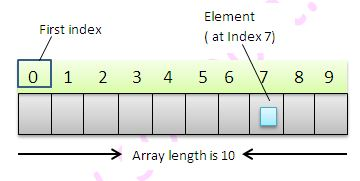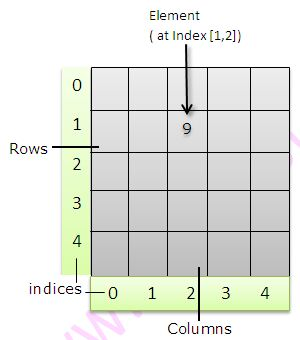Arrays are used to store similar types of elements in sequential manner. Arrays are fixed in size. You can not grow or shrink the size of array once initialized. Example –
``````
int [] a = {11,22,33}

Student [] studentArray = {s1,s2,s3}
``````
There are 3 types of arrays in Java.
• Single Dimensional
• 2-Dimensional
• Multi-dimensional

### Single dimensional Array### Two dimensional ArrayBelow example explains how to use arrays in Java.
``````
package arrays;

/**
* Created by ssalunke on 18/04/2016.
*/
public class Array {

public static void main(String [] args){

System.out.println("Let us learn One dimensional array");

int[] primitiveIntArray = new int;
int[] primitiveInitializedIntArray = {1,2,3};
int[] primitiveInitializedIntArray1 = new int[]{1,2,3};

//Just like primitives, we can store the reference
// types of objects in Arrays
Student s1 = new Student("s1");
Student s2 = new Student("s2");
Student s3 = new Student("s3");

Student[] studentArray = new Student;
Student[] studentInitializedIntArray = {s1,s2,s3};
Student[] studentInitializedIntArray1 = new Student[]{s1,s2,s3};

//Accessing elements in array by using for loop
for(int i=0;i<=2;i++){
System.out.println("Integer Array -> "
+ primitiveInitializedIntArray[i]);
System.out.println("Student Array -> "
+ studentInitializedIntArray[i]);
}

//Accessing elements in array using another variation of for loop
for(int e: primitiveInitializedIntArray){
System.out.println("Integer Array -> " + e );
}
for(Student e: studentInitializedIntArray){
System.out.println("Student Array -> " + e );
}

//***************************************************************//
//Learning 2 dimensional arrays
int [][] twoDimensionalIntArray = new int;
for(int i=0;i<=2;i++)
for(int j=0;j<=2;j++)
{
twoDimensionalIntArray[i][j] = i+j;
}

for(int[] e: twoDimensionalIntArray){
for(int j:e)
System.out.println("2-D Integer Array -> " + j);
}

//Array of Arrays in Java
int[][] intArrayOfArray =
{primitiveInitializedIntArray,primitiveInitializedIntArray1};
int[][][] intArrayOf2DArray =
{twoDimensionalIntArray,twoDimensionalIntArray};

System.out.println("1st Element in intArrayOfArray -> "
+ intArrayOfArray);
System.out.println("1st Element in intArrayOf2DArray -> "
+ intArrayOf2DArray);

}

}

class Student{
String name;

Student(String s){
this.name = s;
}

@Override
public String toString(){
return this.name;
}
}
``````
Here is the output of above example.
``````
Let us learn One dimensional array
Integer Array -> 1
Student Array -> s1
Integer Array -> 2
Student Array -> s2
Integer Array -> 3
Student Array -> s3
Integer Array -> 1
Integer Array -> 2
Integer Array -> 3
Student Array -> s1
Student Array -> s2
Student Array -> s3
2-D Integer Array -> 0
2-D Integer Array -> 1
2-D Integer Array -> 2
2-D Integer Array -> 1
2-D Integer Array -> 2
2-D Integer Array -> 3
2-D Integer Array -> 2
2-D Integer Array -> 3
2-D Integer Array -> 4
1st Element in intArrayOfArray -> 1
1st Element in intArrayOf2DArray -> 0
``````
Complex problems, Simple Solutions